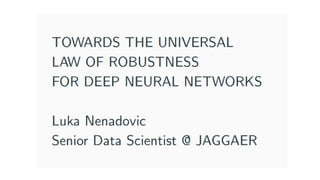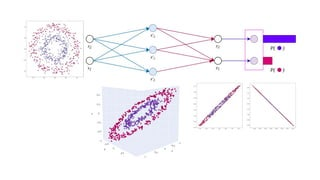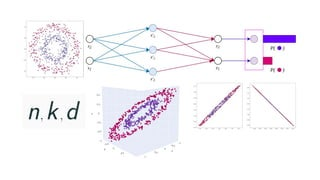Successfully reported this slideshow.

# [DSC Europe 22] Towards the Universal Law of Robustness for Deep Neural Networks - Luka Nenadovic×

# [DSC Europe 22] Towards the Universal Law of Robustness for Deep Neural Networks - Luka Nenadovic

We will present some recent fundamental developments in understanding how neural networks work, in particular why the overparametrized models with the billions or even trilions of parameters perform so well even in cases when the number of parameters outnumbers the number of training samples, contrary to classical machine learning paradigm. We will present the BLN Conjecture (2020) and demonstrate few extreme cases of Bubeck-Sellke Theorem (2021). In order to illustrate highly technical theorem and it's proof we will use an original pictorial approach to represent the behaviour of neural networks from the point of view of the higher-dimensional geometry.

We will present some recent fundamental developments in understanding how neural networks work, in particular why the overparametrized models with the billions or even trilions of parameters perform so well even in cases when the number of parameters outnumbers the number of training samples, contrary to classical machine learning paradigm. We will present the BLN Conjecture (2020) and demonstrate few extreme cases of Bubeck-Sellke Theorem (2021). In order to illustrate highly technical theorem and it's proof we will use an original pictorial approach to represent the behaviour of neural networks from the point of view of the higher-dimensional geometry.

### [DSC Europe 22] Towards the Universal Law of Robustness for Deep Neural Networks - Luka Nenadovic

1. 1. Sketch of the proof:
2. 2. Sketch of the proof:
3. 3. Sketch of the proof:
4. 4. Sketch of the proof (d=2):
5. 5. Sketch of the proof (d=2):
6. 6. Sketch of the proof (d=2):
7. 7. Sketch of the proof (d=2):
8. 8. Sketch of the proof (d=2):
9. 9. Sketch of the proof (d=2):
10. 10. Sketch of the proof (d=2):
11. 11. Over-parametrization is necessary for robustness!
12. 12. Over-parametrization is necessary for robustness!
13. 13. Over-parametrization is necessary for robustness!
14. 14. Curse of dimensionality!
15. 15. This is huge over-parametrization!
16. 16. This is huge over-parametrization!
17. 17. This is huge over-parametrization!
18. 18. Source: nilesjohnson.net
19. 19. •Thank you!